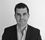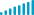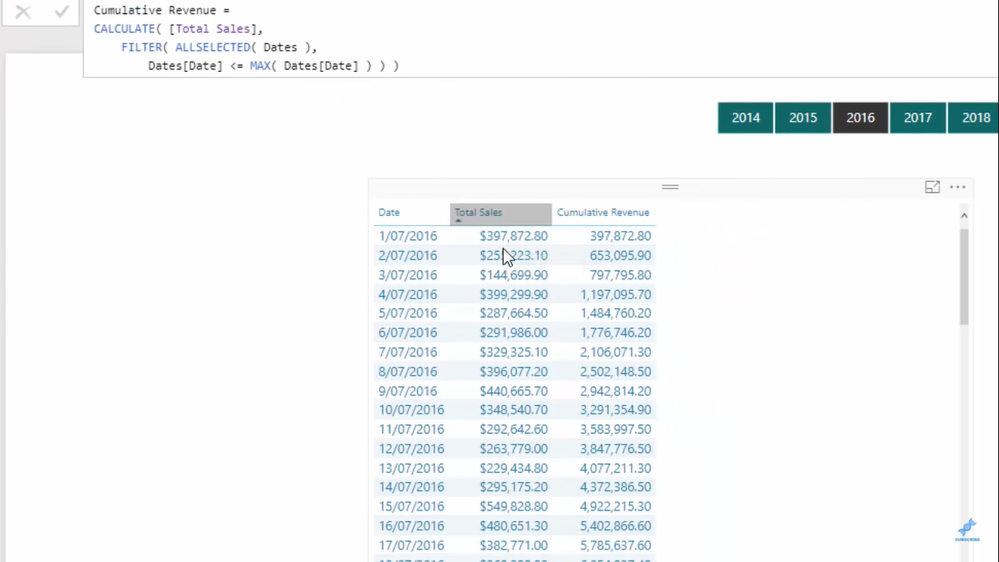cancel
Showing results for
Did you mean:## The CALCULATE Function In Power BI - DAX Tutorial

In this blog, I’ll cover how the CALCULATE function works and why it’s a very significant DAX function in Power BI. I’ll show you some examples of its application through some of our tutorials on Enterprise DNA.

You can easily branch out to time intelligence calculations by using the CALCULATE function. Once you apply this technique, you’ll be able to produce high quality insights inside Power BI.

You’ll see in this first tutorial how CALCULATE is being used in a time intelligence calculation. In this example, CALCULATE is used together with SAMPERIODLASTYEAR to compare the sales on the current date to the sales on the same date last year.Watch the full video below and see how you could use CALCULATE to change, adjust, or add to the context of your time-related calculations.

The key thing to understand about the CALCULATE function is that it changes the context of your calculation in Power BI. It’s quite useful when you run some time intelligence calculation where you need to change the context. And so, to truly understand this, it’s also important that you get a good grasp of row and filter context as well.This next tutorial simplifies the key ideas around row and filter context to help you better understand the use of the CALCULATE function. Here’s the full video.

Lastly, I want to show you how CALCULATE is used in running totals or cumulative totals. Running total is the perfect way to display patterns and changes on a specified data over time.In Power BI, there is a common combination of DAX functions that allow us to create a dynamic cumulative total on any report page. And the key DAX function here is CALCULATE. Once you understand how CALCULATE works in this formula pattern, you’ll understand the logic for calculating running totals, and it’ll be easier for you to use it in different ways.

Here’s the full video tutorial.

Key Take Away

The CALCULATE function allows you to change the context of a calculation within a measure wherein the context is coming from the environment where your calculation is being done. The ability to change the context within a measure is very important in DAX. Hence, CALCULATE is a very important DAX function in Power BI.

The CALCULATE function enables you to work in different ways using a similar insight without having to rewrite formulas or calculations. CALCULATE will become your most used function since there are many ways that you can adjust the context.

Think about how you can apply this on something that you are working on. If you can utilize this function, you can quickly do formula combinations and techniques to calculate more things.

All the best!

Sam

***** Related Course Modules*****

***** Related Support Forum Posts *****